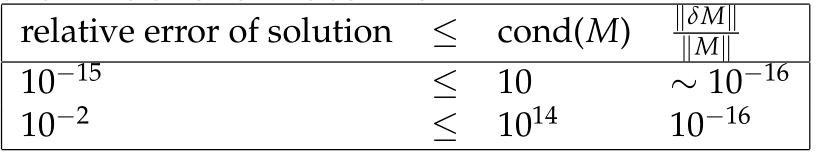## ECE1505H Convex Optimization. Lecture 3: Matrix functions, SVD, and types of Sets. Taught by Prof. Stark Draper

### Disclaimer

Peeter’s lecture notes from class. These may be incoherent and rough.

These are notes for the UofT course ECE1505H, Convex Optimization, taught by Prof. Stark Draper.

## Matrix inner product

Given real matrices $$X, Y \in \mathbb{R}^{m\times n}$$, one possible matrix inner product definition is

\begin{equation}\label{eqn:convexOptimizationLecture3:20}
\begin{aligned}
\innerprod{X}{Y}
&= \textrm{Tr}( X^\T Y) \\
&= \textrm{Tr} \lr{ \sum_{k = 1}^m X_{ki} Y_{kj} } \\
&= \sum_{k = 1}^m \sum_{j = 1}^n X_{kj} Y_{kj} \\
&= \sum_{i = 1}^m \sum_{j = 1}^n X_{ij} Y_{ij}.
\end{aligned}
\end{equation}

This inner product induces a norm on the (matrix) vector space, called the Frobenius norm

\begin{equation}\label{eqn:convexOptimizationLecture3:40}
\begin{aligned}
\Norm{X }_F
&= \textrm{Tr}( X^\T X) \\
&= \sqrt{ \innerprod{X}{X} } \\
&=
\sum_{i = 1}^m \sum_{j = 1}^n X_{ij}^2.
\end{aligned}
\end{equation}

## Range, nullspace.

Definition: Range: Given $$A \in \mathbb{R}^{m \times n}$$, the range of A is the set:

\begin{equation*}
\mathcal{R}(A) = \setlr{ A \Bx | \Bx \in \mathbb{R}^n }.
\end{equation*}

Definition: Nullspace: Given $$A \in \mathbb{R}^{m \times n}$$, the nullspace of A is the set:

\begin{equation*}
\mathcal{N}(A) = \setlr{ \Bx | A \Bx = 0 }.
\end{equation*}

## SVD.

To understand operation of $$A \in \mathbb{R}^{m \times n}$$, a representation of a linear transformation from \R{n} to \R{m}, decompose $$A$$ using the singular value decomposition (SVD).

Definition: SVD: Given $$A \in \mathbb{R}^{m \times n}$$, an operator on $$\Bx \in \mathbb{R}^n$$, a decomposition of the following form is always possible

\begin{equation*}
\begin{aligned}
A &= U \Sigma V^\T \\
U &\in \mathbb{R}^{m \times r} \\
V &\in \mathbb{R}^{n \times r},
\end{aligned}
\end{equation*}

where $$r$$ is the rank of $$A$$, and both $$U$$ and $$V$$ are orthogonal

\begin{equation*}
\begin{aligned}
U^\T U &= I \in \mathbb{R}^{r \times r} \\
V^\T V &= I \in \mathbb{R}^{r \times r}.
\end{aligned}
\end{equation*}

Here $$\Sigma = \textrm{diag}( \sigma_1, \sigma_2, \cdots, \sigma_r )$$, is a diagonal matrix of “singular” values, where

\begin{equation*}
\sigma_1 \ge \sigma_2 \ge \cdots \ge \sigma_r.
\end{equation*}

For simplicity consider square case $$m = n$$

\begin{equation}\label{eqn:convexOptimizationLecture3:100}
A \Bx = \lr{ U \Sigma V^\T } \Bx.
\end{equation}

The first product $$V^\T \Bx$$ is a rotation, which can be checked by looking at the length

\begin{equation}\label{eqn:convexOptimizationLecture3:120}
\begin{aligned}
\Norm{ V^\T \Bx}_2
&= \sqrt{ \Bx^\T V V^\T \Bx } \\
&= \sqrt{ \Bx^\T \Bx } \\
&= \Norm{ \Bx }_2,
\end{aligned}
\end{equation}

which shows that the length of the vector is unchanged after application of the linear transformation represented by $$V^\T$$ so that operation must be a rotation.

Similarly the operation of $$U$$ on $$\Sigma V^\T \Bx$$ also must be a rotation. The operation $$\Sigma = [\sigma_i]_i$$ applies a scaling operation to each component of the vector $$V^\T \Bx$$.

All linear (square) transformations can therefore be thought of as a rotate-scale-rotate operation. Often the $$A$$ of interest will be symmetric $$A = A^\T$$.

## Set of symmetric matrices

Let $$S^n$$ be the set of real, symmetric $$n \times n$$ matrices.

Theorem: Spectral theorem: When $$A \in S^n$$ then it is possible to factor $$A$$ as

\begin{equation*}
A = Q \Lambda Q^\T,
\end{equation*}

where $$Q$$ is an orthogonal matrix, and $$\Lambda = \textrm{diag}( \lambda_1, \lambda_2, \cdots \lambda_n)$$. Here $$\lambda_i \in \mathbb{R} \, \forall i$$ are the (real) eigenvalues of $$A$$.

A real symmetric matrix $$A \in S^n$$ is “positive semi-definite” if

\begin{equation*}
\Bv^\T A \Bv \ge 0 \qquad\forall \Bv \in \mathbb{R}^n, \Bv \ne 0,
\end{equation*}
and is “positive definite” if

\begin{equation*}
\Bv^\T A \Bv > 0 \qquad\forall \Bv \in \mathbb{R}^n, \Bv \ne 0.
\end{equation*}

The set of such matrices is denoted $$S^n_{+}$$, and $$S^n_{++}$$ respectively.

Consider $$A \in S^n_{+}$$ (or $$S^n_{++}$$ )

\begin{equation}\label{eqn:convexOptimizationLecture3:200}
A = Q \Lambda Q^\T,
\end{equation}

possible since the matrix is symmetric. For such a matrix

\begin{equation}\label{eqn:convexOptimizationLecture3:220}
\begin{aligned}
\Bv^\T A \Bv
&=
\Bv^\T Q \Lambda A^\T \Bv \\
&=
\Bw^\T \Lambda \Bw,
\end{aligned}
\end{equation}

where $$\Bw = A^\T \Bv$$. Such a product is

\begin{equation}\label{eqn:convexOptimizationLecture3:240}
\Bv^\T A \Bv
=
\sum_{i = 1}^n \lambda_i w_i^2.
\end{equation}

So, if $$\lambda_i \ge 0$$ ($$\lambda_i > 0$$ ) then $$\sum_{i = 1}^n \lambda_i w_i^2$$ is non-negative (positive) $$\forall \Bw \in \mathbb{R}^n, \Bw \ne 0$$. Since $$\Bw$$ is just a rotated version of $$\Bv$$ this also holds for all $$\Bv$$. A necessary and sufficient condition for $$A \in S^n_{+}$$ ($$S^n_{++}$$ ) is $$\lambda_i \ge 0$$ ($$\lambda_i > 0$$).

## Square root of positive semi-definite matrix

Real symmetric matrix power relationships such as

\begin{equation}\label{eqn:convexOptimizationLecture3:260}
A^2
=
Q \Lambda Q^\T
Q \Lambda Q^\T
=
Q \Lambda^2
Q^\T
,
\end{equation}

or more generally $$A^k = Q \Lambda^k Q^\T,\, k \in \mathbb{Z}$$, can be further generalized to non-integral powers. In particular, the square root (non-unique) of a square matrix can be written

\begin{equation}\label{eqn:convexOptimizationLecture3:280}
A^{1/2} = Q
\begin{bmatrix}
\sqrt{\lambda_1} & & & \\
& \sqrt{\lambda_2} & & \\
& & \ddots & \\
& & & \sqrt{\lambda_n} \\
\end{bmatrix}
Q^\T,
\end{equation}

since $$A^{1/2} A^{1/2} = A$$, regardless of the sign picked for the square roots in question.

## Functions of matrices

Consider $$F : S^n \rightarrow \mathbb{R}$$, and define

\begin{equation}\label{eqn:convexOptimizationLecture3:300}
F(X) = \log \det X,
\end{equation}

Here $$\textrm{dom} F = S^n_{++}$$. The task is to find $$\spacegrad F$$, which can be done by looking at the perturbation $$\log \det ( X + \Delta X )$$

\begin{equation}\label{eqn:convexOptimizationLecture3:320}
\begin{aligned}
\log \det ( X + \Delta X )
&=
\log \det ( X^{1/2} (I + X^{-1/2} \Delta X X^{-1/2}) X^{1/2} ) \\
&=
\log \det ( X (I + X^{-1/2} \Delta X X^{-1/2}) ) \\
&=
\log \det X + \log \det (I + X^{-1/2} \Delta X X^{-1/2}).
\end{aligned}
\end{equation}

Let $$X^{-1/2} \Delta X X^{-1/2} = M$$ where $$\lambda_i$$ are the eigenvalues of $$M : M \Bv = \lambda_i \Bv$$ when $$\Bv$$ is an eigenvector of $$M$$. In particular

\begin{equation}\label{eqn:convexOptimizationLecture3:340}
(I + M) \Bv =
(1 + \lambda_i) \Bv,
\end{equation}

where $$1 + \lambda_i$$ are the eigenvalues of the $$I + M$$ matrix. Since the determinant is the product of the eigenvalues, this gives

\begin{equation}\label{eqn:convexOptimizationLecture3:360}
\begin{aligned}
\log \det ( X + \Delta X )
&=
\log \det X +
\log \prod_{i = 1}^n (1 + \lambda_i) \\
&=
\log \det X +
\sum_{i = 1}^n \log (1 + \lambda_i).
\end{aligned}
\end{equation}

If $$\lambda_i$$ are sufficiently “small”, then $$\log ( 1 + \lambda_i ) \approx \lambda_i$$, giving

\begin{equation}\label{eqn:convexOptimizationLecture3:380}
\log \det ( X + \Delta X )
=
\log \det X +
\sum_{i = 1}^n \lambda_i
\approx
\log \det X +
\textrm{Tr}( X^{-1/2} \Delta X X^{-1/2} ).
\end{equation}

Since
\begin{equation}\label{eqn:convexOptimizationLecture3:400}
\textrm{Tr}( A B ) = \textrm{Tr}( B A ),
\end{equation}

this trace operation can be written as

\begin{equation}\label{eqn:convexOptimizationLecture3:420}
\log \det ( X + \Delta X )
\approx
\log \det X +
\textrm{Tr}( X^{-1} \Delta X )
=
\log \det X +
\innerprod{ X^{-1}}{\Delta X},
\end{equation}

so
\begin{equation}\label{eqn:convexOptimizationLecture3:440}
\end{equation}

To check this, consider the simplest example with $$X \in \mathbb{R}^{1 \times 1}$$, where we have

\begin{equation}\label{eqn:convexOptimizationLecture3:460}
\frac{d}{dX} \lr{ \log \det X } = \frac{d}{dX} \lr{ \log X } = \inv{X} = X^{-1}.
\end{equation}

This is a nice example demonstrating how the gradient can be obtained by performing a first order perturbation of the function. The gradient can then be read off from the result.

## Second order perturbations

• To get first order approximation found the part that varied linearly in $$\Delta X$$.
• To get the second order part, perturb $$X^{-1}$$ by $$\Delta X$$ and see how that perturbation varies in $$\Delta X$$.

For $$G(X) = X^{-1}$$, this is

\begin{equation}\label{eqn:convexOptimizationLecture3:480}
\begin{aligned}
(X + \Delta X)^{-1}
&=
\lr{ X^{1/2} (I + X^{-1/2} \Delta X X^{-1/2} ) X^{1/2} }^{-1} \\
&=
X^{-1/2} (I + X^{-1/2} \Delta X X^{-1/2} )^{-1} X^{-1/2}
\end{aligned}
\end{equation}

To be proven in the homework (for “small” A)

\begin{equation}\label{eqn:convexOptimizationLecture3:500}
(I + A)^{-1} \approx I – A.
\end{equation}

This gives

\begin{equation}\label{eqn:convexOptimizationLecture3:520}
\begin{aligned}
(X + \Delta X)^{-1}
&=
X^{-1/2} (I – X^{-1/2} \Delta X X^{-1/2} ) X^{-1/2} \\
&=
X^{-1} – X^{-1} \Delta X X^{-1},
\end{aligned}
\end{equation}

or

\begin{equation}\label{eqn:convexOptimizationLecture3:800}
\begin{aligned}
G(X + \Delta X)
&= G(X) + (D G) \Delta X \\
&= G(X) + (\spacegrad G)^\T \Delta X,
\end{aligned}
\end{equation}

so
\begin{equation}\label{eqn:convexOptimizationLecture3:820}
=
– X^{-1} \Delta X X^{-1}.
\end{equation}

The Taylor expansion of $$F$$ to second order is

\begin{equation}\label{eqn:convexOptimizationLecture3:840}
F(X + \Delta X)
=
F(X)
+
\textrm{Tr} \lr{ (\spacegrad F)^\T \Delta X}
+
\inv{2}
\lr{ (\Delta X)^\T (\spacegrad^2 F) \Delta X}.
\end{equation}

The first trace can be expressed as an inner product

\begin{equation}\label{eqn:convexOptimizationLecture3:860}
\begin{aligned}
\textrm{Tr} \lr{ (\spacegrad F)^\T \Delta X}
&=
\innerprod{ \spacegrad F }{\Delta X} \\
&=
\innerprod{ X^{-1} }{\Delta X}.
\end{aligned}
\end{equation}

The second trace also has the structure of an inner product

\begin{equation}\label{eqn:convexOptimizationLecture3:880}
\begin{aligned}
(\Delta X)^\T (\spacegrad^2 F) \Delta X
&=
\textrm{Tr} \lr{ (\Delta X)^\T (\spacegrad^2 F) \Delta X} \\
&=
\innerprod{ (\spacegrad^2 F)^\T \Delta X }{\Delta X},
\end{aligned}
\end{equation}

where a no-op trace could be inserted in the second order term since that quadratic form is already a scalar. This $$(\spacegrad^2 F)^\T \Delta X$$ term has essentially been found implicitly by performing the linear variation of $$\spacegrad F$$ in $$\Delta X$$, showing that we must have

\begin{equation}\label{eqn:convexOptimizationLecture3:900}
\textrm{Tr} \lr{ (\Delta X)^\T (\spacegrad^2 F) \Delta X}
=
\innerprod{ – X^{-1} \Delta X X^{-1} }{\Delta X},
\end{equation}

so
\begin{equation}\label{eqn:convexOptimizationLecture3:560}
F( X + \Delta X) = F(X) +
\innerprod{X^{-1}}{\Delta X}
+\inv{2} \innerprod{-X^{-1} \Delta X X^{-1}}{\Delta X},
\end{equation}

or
\begin{equation}\label{eqn:convexOptimizationLecture3:580}
\log \det ( X + \Delta X) = \log \det X +
\textrm{Tr}( X^{-1} \Delta X )
– \inv{2} \textrm{Tr}( X^{-1} \Delta X X^{-1} \Delta X ).
\end{equation}

## Convex Sets

• Types of sets: Affine, convex, cones
• Examples: Hyperplanes, polyhedra, balls, ellipses, norm balls, cone of PSD matrices.

Definition: Affine set:

A set $$C \subseteq \mathbb{R}^n$$ is affine if $$\forall \Bx_1, \Bx_2 \in C$$ then

\begin{equation*}
\theta \Bx_1 + (1 -\theta) \Bx_2 \in C, \qquad \forall \theta \in \mathbb{R}.
\end{equation*}

The affine sum above can
be rewritten as

\begin{equation}\label{eqn:convexOptimizationLecture3:600}
\Bx_2 + \theta (\Bx_1 – \Bx_2).
\end{equation}

Since $$\theta$$ is a scaling, this is the line containing $$\Bx_2$$ in the direction between $$\Bx_1$$ and $$\Bx_2$$.

Observe that the solution to a set of linear equations

\begin{equation}\label{eqn:convexOptimizationLecture3:620}
C = \setlr{ \Bx | A \Bx = \Bb },
\end{equation}

is an affine set. To check, note that

\begin{equation}\label{eqn:convexOptimizationLecture3:640}
\begin{aligned}
A (\theta \Bx_1 + (1 – \theta) \Bx_2)
&=
\theta A \Bx_1 + (1 – \theta) A \Bx_2 \\
&=
\theta \Bb + (1 – \theta) \Bb \\
&= \Bb.
\end{aligned}
\end{equation}

Definition: Affine combination: An affine combination of points $$\Bx_1, \Bx_2, \cdots \Bx_n$$ is

\begin{equation*}
\sum_{i = 1}^n \theta_i \Bx_i,
\end{equation*}

such that for $$\theta_i \in \mathbb{R}$$

\begin{equation*}
\sum_{i = 1}^n \theta_i = 1.
\end{equation*}

An affine set contains all affine combinations of points in the set. Examples of a couple affine sets are sketched in fig 1.1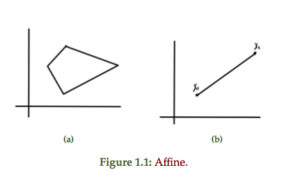For comparison, a couple of non-affine sets are sketched in fig 1.2Definition: Convex set: A set $$C \subseteq \mathbb{R}^n$$ is convex if $$\forall \Bx_1, \Bx_2 \in C$$ and $$\forall \theta \in \mathbb{R}, \theta \in [0,1]$$, the combination

\begin{equation}\label{eqn:convexOptimizationLecture3:700}
\theta \Bx_1 + (1 – \theta) \Bx_2 \in C.
\end{equation}

Definition: Convex combination: A convex combination of $$\Bx_1, \Bx_2, \cdots \Bx_n$$ is

\begin{equation*}
\sum_{i = 1}^n \theta_i \Bx_i,
\end{equation*}

such that $$\forall \theta_i \ge 0$$

\begin{equation*}
\sum_{i = 1}^n \theta_i = 1
\end{equation*}

Definition: Convex hull: Convex hull of a set $$C$$ is a set of all convex combinations of points in $$C$$, denoted

\begin{equation}\label{eqn:convexOptimizationLecture3:720}
\textrm{conv}(C) = \setlr{ \sum_{i=1}^n \theta_i \Bx_i | \Bx_i \in C, \theta_i \ge 0, \sum_{i=1}^n \theta_i = 1 }.
\end{equation}

A non-convex set can be converted into a convex hull by filling in all the combinations of points connecting points in the set, as sketched in fig 1.3.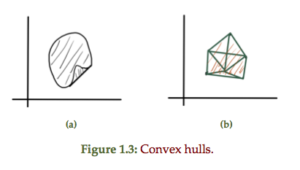Definition: Cone: A set $$C$$ is a cone if $$\forall \Bx \in C$$ and $$\forall \theta \ge 0$$ we have $$\theta \Bx \in C$$.

This scales out if $$\theta > 1$$ and scales in if $$\theta < 1$$.

A convex cone is a cone that is also a convex set. A conic combination is

\begin{equation*}
\sum_{i=1}^n \theta_i \Bx_i, \theta_i \ge 0.
\end{equation*}

A convex and non-convex 2D cone is sketched in fig. 1.4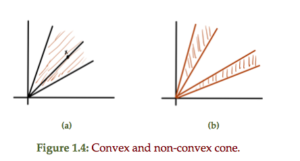A comparison of properties for different set types is tabulated in table 1.1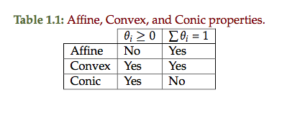## Hyperplanes and half spaces

Definition: Hyperplane: A hyperplane is defined by

\begin{equation*}
\setlr{ \Bx | \Ba^\T \Bx = \Bb, \Ba \ne 0 }.
\end{equation*}

A line and plane are examples of this general construct as sketched in
fig. 1.5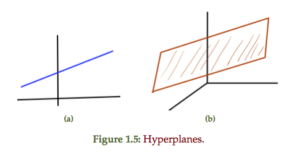An alternate view is possible should one
find any specific $$\Bx_0$$ such that $$\Ba^\T \Bx_0 = \Bb$$

\begin{equation}\label{eqn:convexOptimizationLecture3:740}
\setlr{\Bx | \Ba^\T \Bx = b }
=
\setlr{\Bx | \Ba^\T (\Bx -\Bx_0) = 0 }
\end{equation}

This shows that $$\Bx – \Bx_0 = \Ba^\perp$$ is perpendicular to $$\Ba$$, or

\begin{equation}\label{eqn:convexOptimizationLecture3:780}
\Bx
=
\Bx_0 + \Ba^\perp.
\end{equation}

This is the subspace perpendicular to $$\Ba$$ shifted by $$\Bx_0$$, subject to $$\Ba^\T \Bx_0 = \Bb$$. As a set

\begin{equation}\label{eqn:convexOptimizationLecture3:760}
\Ba^\perp = \setlr{ \Bv | \Ba^\T \Bv = 0 }.
\end{equation}

## Half space

Definition: Half space: The half space is defined as
\begin{equation*}
\setlr{ \Bx | \Ba^\T \Bx = \Bb }
= \setlr{ \Bx | \Ba^\T (\Bx – \Bx_0) \le 0 }.
\end{equation*}

This can also be expressed as $$\setlr{ \Bx | \innerprod{ \Ba }{\Bx – \Bx_0 } \le 0 }$$.

## Final notes for ECE1254, Modelling of Multiphysics Systems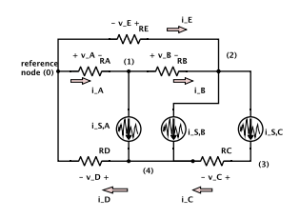I’ve now finished my first grad course, Modelling of Multiphysics Systems, taught by Prof Piero Triverio.

I’ve posted notes for lectures and other material as I was taking the course, but now have an aggregated set of notes for the whole course posted.
This is now updated with all my notes from the lectures, solved problems, additional notes on auxillary topics I wanted to explore (like SVD), plus the notes from the Harmonic Balance report that Mike and I will be presenting in January.

This version of my notes also includes all the matlab figures regenerating using http://www.mathworks.com/matlabcentral/fileexchange/23629-export-fig, which allows a save-as pdf, which rescales much better than Matlab saveas() png’s when embedded in latex.  I’m not sure if that’s the best way to include Matlab figures in latex, but they are at least not fuzzy looking now.

All in all, I’m pretty pleased with my notes for this course.  They are a lot more readable than any of the ones I’ve done for the physics undergrad courses I was taking (http://peeterjoot.com/writing/).  While there was quite a lot covered in this course, the material really only requires an introductory circuits course and some basic math (linear algebra and intro calculus), so is pretty accessible.

This was a fun course.  I recall, back in ancient times when I was a first year student, being unsatisfied with all the ad-hoc strategies we used to solve circuits problems.  This finally answers the questions of how to tackle things more systematically.

Here’s the contents outline for these notes:

Preface
Lecture notes
1 nodal analysis
1.1 In slides
1.2 Mechanical structures example
1.3 Assembling system equations automatically. Node/branch method
1.4 Nodal Analysis
1.5 Modified nodal analysis (MNA)
2 solving large systems
2.1 Gaussian elimination
2.2 LU decomposition
2.3 Problems
3 numerical errors and conditioning
3.1 Strict diagonal dominance
3.2 Exploring uniqueness and existence
3.3 Perturbation and norms
3.4 Matrix norm
4 singular value decomposition, and conditioning number
4.1 Singular value decomposition
4.2 Conditioning number
5 sparse factorization
5.1 Fill ins
5.2 Markowitz product
5.3 Markowitz reordering
5.4 Graph representation
6.1 Summary of factorization costs
6.2 Iterative methods
6.4 Recap: Summary of Gradient method
6.6 Full Algorithm
6.7 Order analysis
6.9 Gershgorin circle theorem
6.10 Preconditioning
6.11 Symmetric preconditioning
6.13 Problems
7 solution of nonlinear systems
7.1 Nonlinear systems
7.2 Richardson and Linear Convergence
7.3 Newton’s method
7.4 Solution of N nonlinear equations in N unknowns
7.5 Multivariable Newton’s iteration
7.6 Automatic assembly of equations for nonlinear system
7.7 Damped Newton’s method
7.8 Continuation parameters
7.9 Singular Jacobians
7.10 Struts and Joints, Node branch formulation
7.11 Problems
8 time dependent systems
8.1 Assembling equations automatically for dynamical systems
8.2 Numerical solution of differential equations
8.3 Forward Euler method
8.4 Backward Euler method
8.5 Trapezoidal rule (TR)
8.6 Nonlinear differential equations
8.7 Analysis, accuracy and stability (Dt ! 0)
8.8 Residual for LMS methods
8.9 Global error estimate
8.10 Stability
8.11 Stability (continued)
8.12 Problems
9 model order reduction
9.1 Model order reduction
9.2 Moment matching
9.3 Model order reduction (cont).
9.4 Moment matching
9.5 Truncated Balanced Realization (1000 ft overview)
9.6 Problems
Final report
10 harmonic balance
10.1 Abstract
10.2 Introduction
10.2.1 Modifications to the netlist syntax
10.3 Background
10.3.1 Discrete Fourier Transform
10.3.2 Harmonic Balance equations
10.3.3 Frequency domain representation of MNA equations
10.3.4 Example. RC circuit with a diode.
10.3.5 Jacobian
10.3.6 Newton’s method solution
10.3.7 Alternative handling of the non-linear currents and Jacobians
10.4 Results
10.4.1 Low pass filter
10.4.2 Half wave rectifier
10.4.3 AC to DC conversion
10.4.4 Bridge rectifier
10.4.5 Cpu time and error vs N
10.4.6 Taylor series non-linearities
10.4.7 Stiff systems
10.5 Conclusion
10.6 Appendices
10.6.1 Discrete Fourier Transform inversion
Appendices
a singular value decomposition
b basic theorems and definitions
c norton equivalents
d stability of discretized linear differential equations
e laplace transform refresher
f discrete fourier transform
g harmonic balance, rough notes
g.1 Block matrix form, with physical parameter ordering
g.2 Block matrix form, with frequency ordering
g.3 Representing the linear sources
g.4 Representing non-linear sources
g.5 Newton’s method
g.6 A matrix formulation of Harmonic Balance non-linear currents
h matlab notebooks
i mathematica notebooks
Index
Bibliography

## Singular Value Decomposition

October 2, 2014 ece1254 , , , ,

We’ve been presented with the definition of the singular value decomposition or SVD, but not how to compute it.

Recall that the definition was

## Singular value decomposition (SVD)

Given $$M \in \text{R}^{m \times n}$$, we can find a representation of $$M$$

\begin{equation}\label{eqn:svdNotes:81}
M = U \Sigma V^\T,
\end{equation}

where $$U$$ and $$V$$ are orthogonal matrices such that $$U^\T U = 1$$, and $$V^\T V = 1$$, and

\begin{equation}\label{eqn:svdNotes:101}
\Sigma =
\begin{bmatrix}
\sigma_1 & & & & & &\\
& \sigma_2 & & & & &\\
& & \ddots & & & &\\
& & & \sigma_r & & &\\
& & & & 0 & & \\
& & & & & \ddots & \\
& & & & & & 0 \\
\end{bmatrix}
\end{equation}

The values $$\sigma_i, i \le \min(n,m)$$ are called the singular values of $$M$$. The singular values are subject to the ordering

\begin{equation}\label{eqn:svdNotes:261}
\sigma_{1} \ge \sigma_{2} \ge \cdots \ge 0
\end{equation}

If $$r$$ is the rank of $$M$$, then the $$\sigma_r$$ above is the minimum non-zero singular value (but the zeros are also called singular values).

Here I’ll review some of the key ideas from the MIT OCW SVD lecture by Prof. Gilbert Strang. This is largely to avoid having to rewatch this again in a few years as I just did.

The idea behind the SVD is to find an orthogonal basis that relates the image space of the transformation, as well as the basis for the vectors that the transformation applies to. That is a relation of the form

\begin{equation}\label{eqn:svdNotes:281}
\Bu_i = M \Bv_j
\end{equation}

Where $$\Bv_j$$ are orthonormal basis vectors, and $$\Bu_i$$ are orthonormal basis vectors for the image space. %Strang’s lectures call these the row and column spaces (or reverse?)

Let’s suppose that such a set of vectors can be computed and that $$M$$ has a representation of the desired form

\begin{equation}\label{eqn:svdNotes:301}
M = U \Sigma V^\conj
\end{equation}

where
\begin{equation}\label{eqn:svdNotes:321}
U =
\begin{bmatrix}
\Bu_1 & \Bu_2 & \cdots & \Bu_m
\end{bmatrix},
\end{equation}

and

\begin{equation}\label{eqn:svdNotes:341}
V =
\begin{bmatrix}
\Bv_1 & \Bv_2 & \cdots & \Bv_n
\end{bmatrix}.
\end{equation}

By left or right multiplication of $$M$$ with its (conjugate) transpose, we will see that the decomposed form of these products have a very simple form

\begin{equation}\label{eqn:svdNotes:361}
M^\conj M
=
V \Sigma^\conj U^\conj U \Sigma V^\conj
=
V \Sigma^\conj \Sigma V^\conj,
\end{equation}
\begin{equation}\label{eqn:svdNotes:381}
M M^\conj
=
U \Sigma V^\conj V \Sigma^\conj U^\conj
=
U \Sigma \Sigma^\conj U^\conj.
\end{equation}

Because $$\Sigma$$ is diagonal, the products $$\Sigma^\conj \Sigma$$ and $$\Sigma \Sigma^\conj$$ are also both diagonal, and populated with the absolute squares of the singular values that we have presumed to exist. Because $$\Sigma \Sigma^\conj$$ is an $$m \times m$$ matrix, whereas $$\Sigma^\conj \Sigma$$ is an $$n \times n$$ matrix, so the numbers of zeros in each of these will differ, but each will have the structure

\begin{equation}\label{eqn:svdNotes:401}
\Sigma^\conj \Sigma \sim \Sigma \Sigma^\conj \sim
\begin{bmatrix}
\Abs{\sigma_1}^2 & & & & & &\\
& \Abs{ \sigma_2 }^2 & & & & &\\
& & \ddots & & & &\\
& & & \Abs{ \sigma_r }^2 & & &\\
& & & & 0 & & \\
& & & & & \ddots & \\
& & & & & & 0 \\
\end{bmatrix}
\end{equation}

This shows us one method of computing the singular value decomposition (for full rank systems), because we need only solve for the eigensystem of either $$M M^\conj$$ or $$M^\conj M$$ to find the singular values, and one of $$U$$ or $$V$$. To form $$U$$ we will be able to find $$r$$ orthonormal eigenvectors of $$M M^\conj$$ and then supplement that with a mutually orthonormal set of vectors from the Null space of $$M$$. We can form $$\Sigma$$ by taking the square roots of the eigenvalues of $$M M^\conj$$. With both $$\Sigma$$ and $$U$$ computed, we can then compute $$V$$ by inversion. We could similarly solve for $$\Sigma$$ and $$V$$ by computing the eigensystem of $$M^\conj M$$ and similarly supplementing those eigenvectors with vectors from the null space, and then compute $$U$$ by inversion.

Let’s work the examples from the lecture to see how this works.

## Example: Full rank 2×2 matrix

In the lecture the SVD decomposition is computed for

\begin{equation}\label{eqn:svdNotes:421}
M =
\begin{bmatrix}
4 & 4 \\
3 & -3
\end{bmatrix}
\end{equation}

For this we have

\begin{equation}\label{eqn:svdNotes:441}
M M^\conj
=
\begin{bmatrix}
32 & 0 \\
0 & 18
\end{bmatrix}
\end{equation}
\begin{equation}\label{eqn:svdNotes:461}
M^\conj M
=
\begin{bmatrix}
25 & 7 \\
7 & 25
\end{bmatrix}
\end{equation}

The first is already diagonalized so $$U = I$$, and the singular values are found by inspection $$\{ \sqrt{32}, \sqrt{18} \}$$, or

\begin{equation}\label{eqn:svdNotes:541}
\Sigma =
\begin{bmatrix}
\sqrt{32} & 0 \\
0 & \sqrt{18}
\end{bmatrix}
\end{equation}

Because the system is full rank we can solve for $$V$$ by inversion

\begin{equation}\label{eqn:svdNotes:481}
\Sigma^{-1} U^\conj M = V^\conj,
\end{equation}

or
\begin{equation}\label{eqn:svdNotes:501}
V = M^\conj U \lr{ \Sigma^{-1} }^\conj.
\end{equation}

In this case that is

\begin{equation}\label{eqn:svdNotes:521}
V = \inv{\sqrt{2}}
\begin{bmatrix}
1 & 1 \\
1 & -1
\end{bmatrix}
\end{equation}

We could alternately compute $$V$$ directly by diagonalizing $$M^\conj M$$. We see that the eigenvectors are $$\lr{ 1, \pm 1 }/\sqrt{2}$$, with respective eigenvalues $$\{ 32, 18 \}$$.

This gives us \ref{eqn:svdNotes:541} and \ref{eqn:svdNotes:521}. Again, because the system is full rank, we can compute $$U$$ by inversion. That is

\begin{equation}\label{eqn:svdNotes:561}
U = M V \Sigma^{-1}.
\end{equation}

Carrying out this calculation recovers $$U = I$$ as expected. Looks like I used a different matrix than Prof. Strang used in his lecture (alternate signs on the 3’s). He had some trouble that arrived from independent calculation of the respective eigenspaces. Calculating one from the other avoids that trouble since there are different signed eigenvalues that can be chosen, and we are looking for specific mappings between the eigenspaces that satisfy the $$\Bu_i = M \Bv_j$$ constraints encoded by the relationship $$M = U \Sigma V^\conj$$.

Let’s work a non-full rank example, as in the lecture.

## Example: 2×2 matrix without full rank

\begin{equation}\label{eqn:svdNotes:581}
M =
\begin{bmatrix}
1 & 1 \\
2 & 2
\end{bmatrix}.
\end{equation}

For this we have

\begin{equation}\label{eqn:svdNotes:601}
M^\conj M =
\begin{bmatrix}
5 & 5 \\
5 & 5
\end{bmatrix}
\end{equation}
\begin{equation}\label{eqn:svdNotes:621}
M M^\conj =
\begin{bmatrix}
2 & 4 \\
4 & 8
\end{bmatrix}
\end{equation}

For which the non-zero eigenvalue is $$10$$ and the corresponding eigenvalue is
\begin{equation}\label{eqn:svdNotes:641}
\Bv =
\inv{\sqrt{2}}
\begin{bmatrix}
1 \\
1
\end{bmatrix}.
\end{equation}

This gives us

\begin{equation}\label{eqn:svdNotes:661}
\Sigma =
\begin{bmatrix}
\sqrt{10} & 0 \\
0 & 0
\end{bmatrix}
\end{equation}

Since we require $$V$$ to be orthonormal, there is only one choice (up to a sign) for the vector from the null space.

Let’s try

\begin{equation}\label{eqn:svdNotes:681}
V =
\inv{\sqrt{2}}
\begin{bmatrix}
1 & 1 \\
1 & -1
\end{bmatrix}
\end{equation}

We find that $$M M^\conj$$ has eigenvalues $$\{ 10, 0 \}$$ as expected. The eigenvector for the non-zero eigenvalue is found to be

\begin{equation}\label{eqn:svdNotes:701}
\Bu = \inv{\sqrt{5}}
\begin{bmatrix}
1 \\
2
\end{bmatrix}.
\end{equation}

It’s easy to expand this to an orthonormal basis, but do we have to pick a specific sign relative to the choice that we’ve made for $$V$$?

Let’s try

\begin{equation}\label{eqn:svdNotes:721}
U = \inv{\sqrt{5}}
\begin{bmatrix}
1 & 2 \\
2 & -1
\end{bmatrix}.
\end{equation}

Multiplying out $$U \Sigma V^\conj$$ we have

\begin{equation}\label{eqn:svdNotes:741}
\begin{aligned}
\inv{\sqrt{5}}
\begin{bmatrix}
1 & 2 \\
2 & -1
\end{bmatrix}
\begin{bmatrix}
\sqrt{10} & 0 \\
0 & 0
\end{bmatrix}
\inv{\sqrt{2}}
\begin{bmatrix}
1 & 1 \\
1 & -1
\end{bmatrix}
&=
\begin{bmatrix}
1 & 2 \\
2 & -1
\end{bmatrix}
\begin{bmatrix}
1 & 0 \\
0 & 0
\end{bmatrix}
\begin{bmatrix}
1 & 1 \\
1 & -1
\end{bmatrix} \\
&=
\begin{bmatrix}
1 & 0 \\
2 & 0
\end{bmatrix}
\begin{bmatrix}
1 & 1 \\
1 & -1
\end{bmatrix} \\
&=
\begin{bmatrix}
1 & 1 \\
2 & 2
\end{bmatrix}.
\end{aligned}
\end{equation}

It appears that this works, although we haven’t demonstrated why that should be, and we could have gotten lucky with signs. There’s some theoretical work to do here, but let’s leave that for another day (or rely on software to do this computational task).

## Disclaimer

Peeter’s lecture notes from class. These may be incoherent and rough.

## Matrix norm

We’ve defined the matrix norm of $$M$$, for the system $$\overline{{y}} = M \overline{{x}}$$ as

\begin{equation}\label{eqn:multiphysicsL6:21}
\Norm{M} = \max_{\Norm{\overline{{x}}} = 1} \Norm{ M \overline{{x}} }.
\end{equation}

We will typically use the $$L_2$$ norm, so that the matrix norm is
\begin{equation}\label{eqn:multiphysicsL6:41}
\Norm{M}_2 = \max_{\Norm{\overline{{x}}}_2 = 1} \Norm{ M \overline{{x}} }_2.
\end{equation}

It can be shown that

\begin{equation}\label{eqn:multiphysicsL6:61}
\Norm{M}_2 = \max_i \sigma_i(M),
\end{equation}

where $$\sigma_i(M)$$ are the (SVD) singular values.

## Singular value decomposition (SVD)

Given $$M \in R^{m \times n}$$, we can find a representation of $$M$$

\begin{equation}\label{eqn:multiphysicsL6:81}
M = U \Sigma V^\T,
\end{equation}

where $$U$$ and $$V$$ are orthogonal matrices such that $$U^\T U = 1$$, and $$V^\T V = 1$$, and

\begin{equation}\label{eqn:multiphysicsL6:101}
\Sigma =
\begin{bmatrix}
\sigma_1 & & & & & &\\
& \sigma_2 & & & & &\\
& & \ddots & & & &\\
& & & \sigma_r & & &\\
& & & & 0 & & \\
& & & & & \ddots & \\
& & & & & & 0 \\
\end{bmatrix}
\end{equation}

The values $$\sigma_i, i \le \min(n,m)$$ are called the singular values of $$M$$. The singular values are subject to the ordering

\begin{equation}\label{eqn:multiphysicsL6:261}
\sigma_{1} \ge \sigma_{2} \ge \cdots \ge 0
\end{equation}

If $$r$$ is the rank of $$M$$, then the $$\sigma_r$$ above is the minimum non-zero singular value (but the zeros are also called singular values).

Observe that the condition $$U^\T U = 1$$ is a statement of orthonormality. In terms of column vectors $$\overline{{u}}_i$$, such a product written out explicitly is

\begin{equation}\label{eqn:multiphysicsL6:301}
\begin{bmatrix}
\overline{{u}}_1^\T \\ \overline{{u}}_2^\T \\ \vdots \\ \overline{{u}}_m^\T
\end{bmatrix}
\begin{bmatrix}
\overline{{u}}_1 & \overline{{u}}_2 & \cdots & \overline{{u}}_m
\end{bmatrix}
=
\begin{bmatrix}
1 & & & \\
& 1 & & \\
& & \ddots & \\
& & & 1
\end{bmatrix}.
\end{equation}

This is both normality $$\overline{{u}}_i^\T \overline{{u}}_i = 1$$, and orthonormality $$\overline{{u}}_i^\T \overline{{u}}_j = 1, i \ne j$$.

## Example: 2 x 2 case

(for column vectors $$\overline{{u}}_i, \overline{{v}}_j$$).

\begin{equation}\label{eqn:multiphysicsL6:281}
M =
\begin{bmatrix}
\overline{{u}}_1 & \overline{{u}}_2
\end{bmatrix}
\begin{bmatrix}
\sigma_1 & \\
& \sigma_2
\end{bmatrix}
\begin{bmatrix}
\overline{{v}}_1^\T \\
\overline{{v}}_2^\T
\end{bmatrix}
\end{equation}

Consider $$\overline{{y}} = M \overline{{x}}$$, and take an $$\overline{{x}}$$ with $$\Norm{\overline{{x}}}_2 = 1$$

Note: I’ve chosen not to sketch what was drawn on the board. See instead the animated gif of the same in \citep{wiki:svd}.

A very nice video treatment of SVD by Prof Gilbert Strang can be found in \citep{ocw:svd}.

## Conditioning number

Given a perturbation of $$M \overline{{x}} = \overline{{b}}$$ to

\begin{equation}\label{eqn:multiphysicsL6:121}
\lr{ M + \delta M }
\lr{ \overline{{x}} + \delta \overline{{x}} } = \overline{{b}},
\end{equation}

or
\begin{equation}\label{eqn:multiphysicsL6:141}
\underbrace{ M \overline{{x}} – \overline{{b}} }_{=0} + \delta M \overline{{x}} + M \delta \overline{{x}} + \delta M \delta \overline{{x}} = 0.
\end{equation}

This gives

\begin{equation}\label{eqn:multiphysicsL6:161}
M \delta \overline{{x}} = – \delta M \overline{{x}} – \delta M \delta \overline{{x}},
\end{equation}

or
\begin{equation}\label{eqn:multiphysicsL6:181}
\delta \overline{{x}} = – M^{-1} \delta M \lr{ \overline{{x}} + \delta \overline{{x}} }.
\end{equation}

Taking norms

\begin{equation}\label{eqn:multiphysicsL6:201}
\Norm{ \delta \overline{{x}}}_2 = \Norm{
M^{-1} \delta M \lr{ \overline{{x}} + \delta \overline{{x}} }
}_2
\le
\Norm{ M^{-1} }_2 \Norm{ \delta M }_2 \Norm{ \overline{{x}} + \delta \overline{{x}} }_2,
\end{equation}

or
\begin{equation}\label{eqn:multiphysicsL6:221}
\underbrace{ \frac{\Norm{ \delta \overline{{x}}}_2 }{ \Norm{ \overline{{x}} + \delta \overline{{x}}}_2 } }_{\text{relative error of solution}}
\le
\underbrace{ \Norm{M}_2 \Norm{M^{-1}}_2 }_{\text{conditioning number of $$M$$}}
\underbrace{ \frac{ \Norm{ \delta M}_2 } {\Norm{M}_2} }_{\text{relative perturbation of $$M$$}}.
\end{equation}

The conditioning number can be shown to be

\begin{equation}\label{eqn:multiphysicsL6:241}
\text{cond}(M) =
\frac
{\sigma_{\mathrm{max}}}
{\sigma_{\mathrm{min}}}
\ge 1
\end{equation}

FIXME: justify.

### sensitivity to conditioning number

Double precision relative rounding errors can be of the order $$10^{-16} \sim 2^{-54}$$, which allows us to gauge the relative error of the solution